# Errata for An Introduction to Modern Econometrics Using Stata

The errata for An Introduction to Modern Econometrics Using Stata are provided below. Click here for an explanation of how to read an erratum. Click here to learn how to determine the printing number of a book.

 (1), (2) Chapter 2, p. 25, first paragraph, second sentence
 For generate or replace, missing values are propagated; any function of missing data produces missing data. For generate or replace, missing values are propagated. Most Stata functions of missing data return missing values. Exceptions include sum(), min(), and max(); see [D] functions for details.
 (1) Chapter 3, p. 45, second paragraph, last sentence
 For quarterly data, S.x generates xt − xt−4, and S2.x generates xt − xt − 8. For quarterly data, S4.x generates xt − xt−4, and S8.x generates xt − xt−8.
 (1),(2),(3),(4) Chapter 3, p. 67, do-file example and paragraph that follows it
  . use http://www.stata-press.com/data/imeus/census2b, clear (Version of census2a for data validation purposes) . duplicates list state Duplicates in terms of state +---------------+ | obs: state | |---------------| | 16 Kansas | | 17 Kansas | +---------------+ . assert r(sum) == 0 assertion is false r(9); end of do-file r(9);  The return item r(sum) is set equal to the total number of duplicate observations found (here, 2), so the identification of duplicates implies that you need to correct the dataset. The duplicates command could also be applied to numeric variables.  . use http://www.stata-press.com/data/imeus/census2b, clear (Version of census2a for data validation purposes) . duplicates list state Duplicates in terms of state +---------------+ | obs: state | |---------------| | 16 Kansas | | 17 Kansas | +---------------+ . duplicates report state Duplicates in terms of state -------------------------------------- copies | observations surplus ----------+--------------------------- 1 | 48 0 2 | 2 1 -------------------------------------- . assert r(unique_value) == r(N) assertion is false r(9); end of do-file r(9);  The return item r(unique_value) is set equal to the number of unique observations found. If that value falls short of the number of observations, r(N), duplicates exist. The identification of duplicates in this supposedly unique identifier implies that the dataset must be altered before its further use. The duplicates command could also be applied to numeric variables to detect the same condition.
 (1) Chapter 4, p. 73, equation (4.5)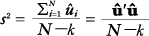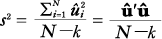(1) Chapter 4, p. 77, unnumbered equation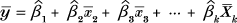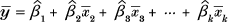(1), (2), (3) Chapter 4, p. 79, first full paragraph after equation, first line
 ... a model, R2 cannot fall and will probably rise, ... a model, R2 cannot fall and will probably rise,
 (1), (2), (3) Chapter 4, p. 79, first full paragraph after equation, fifth line
 zero. Algebraically, ... (N - 1)/(N - k) < 1 for any X zero. Algebraically, ... (N - 1)/(N - k) > 1 for any X
 (1), (2) Chapter 4, p. 85, last paragraph, fourth sentence
 A rule of thumb states that there is evidence of collinearity if the mean VIF is greater than unity or if the largest VIF is greater than 10. A rule of thumb states that there is evidence of collinearity if the largest VIF is greater than 10.
 (1) Chapter 4, p. 96, last paragraph, first sentence
 We cannot reject the hypothesis that the coefficients on ldist and stratio are equal at the 5% level, whereas we can reject the test that the ratio of the lnox and stratio coefficients equals 10 rejected at the 1% level. Whereas we cannot reject the hypothesis that the coefficients on ldist and stratio are equal at the 5% level, we can reject the hypothesis that the ratio of the lnox and stratio coefficients equals 10 at the 1% level.
 (1), (2), (3) Chapter 4, p. 104, second to last unnumbered equation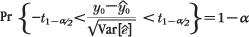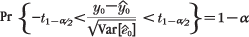(1), (2), (3) Chapter 4, p. 104, last unnumbered equation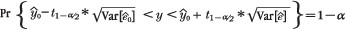(1), (2), (3) Chapter 4, p. 105, top of page
 Plugging in our consistent estimators yields the bounds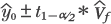Substituting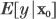for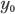and using the variance of predicted value presented in the text yields a prediction interval for the predicted value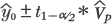Plugging in our consistent estimators yields the bounds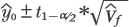Substitutingforand using the variance of predicted value presented in the text yields a prediction interval for the predicted value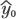.
 (1), (2), (3) Chapter 4, p. 105, second block of Stata code
 . scalar tval = invttail(e(df_r), 0.975) . generate double uplim = xb + tval * stdpred (406 missing values generated) . generate double lowlim = xb - tval * stdpred (406 missing values generated) . scalar tval = invttail(e(df_r), 0.025) . generate double uplim = xb + tval * stdpred (406 missing values generated) . generate double lowlim = xb - tval * stdpred (406 missing values generated)
 (1), (2), (3) Chapter 4, p. 113, equation (4.13)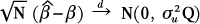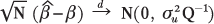(1), (2) Chapter 6, p. 136, equation (6.6)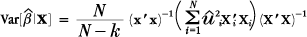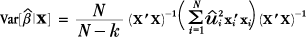(1), (2), (3) Chapter 6, p. 140, first unnumbered equation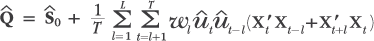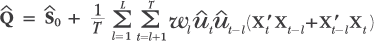(1) Chapter 6, p. 145, equation (6.10)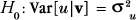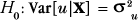(1), (2) Chapter 6, p. 160, Exercise 3, first sentence
 Use the sp500 dataset, applying tsset date. Use the sp500 dataset installed with Stata (sysuse sp500), applying tsset date.
 (1) Chapter 7, p. 176, last paragraph, first sentence
 The deseasonalized series has a smaller standard deviation than the original the seasonality has been removed. The deseasonalized series has a smaller standard deviation than the original as the fraction of the variation due to the seasonality has been removed.
 (1) Chapter 8, p. 196, paragraph after equation 8.10
 For an exactly identified equation, W = iN. For an exactly identified equation, W = IN.
 (1) Chapter 8, p. 196, next paragraph
 Hansen (1982) showed that this process involves choosing W = s-1, where S is the ... Hansen (1982) showed that this process involves choosing W = S-1, where S is the ...
 (1) Chapter 8, p. 196, footnote 10
 Aside from some conditions that guarantee 1/\sqrt(N), z'u is a vector of well-behaved random variables. Aside from some conditions that guarantee 1/\sqrt(N) Z'u is a vector of well-behaved random variables.
 (1), (2) Chapter 9, p. 222, second paragraph, fifth sentence
 De Hoyos and Sarafidis (2006) describe some new tests for contemporaneous correlation, and their command xtscd is available from SSC. De Hoyos and Sarafidis (2006) describe some new tests for contemporaneous correlation, and their command xtcsd is available from SSC.
 (1) Subject index, p. 337, letter M
 maximization likelihood optimization maximum likelihood estimation
 (1), (2) Subject index, p. 341, letter X
 xtscd command xtcsd command